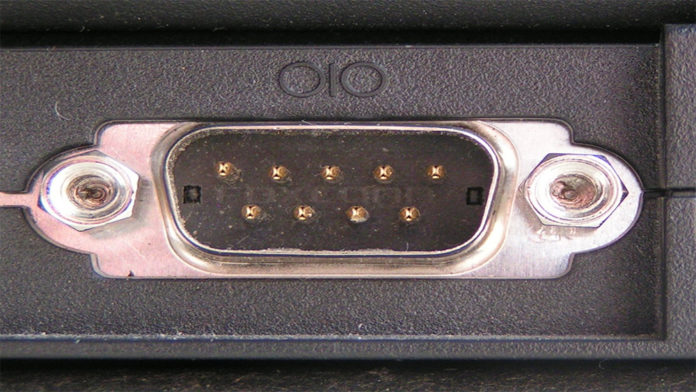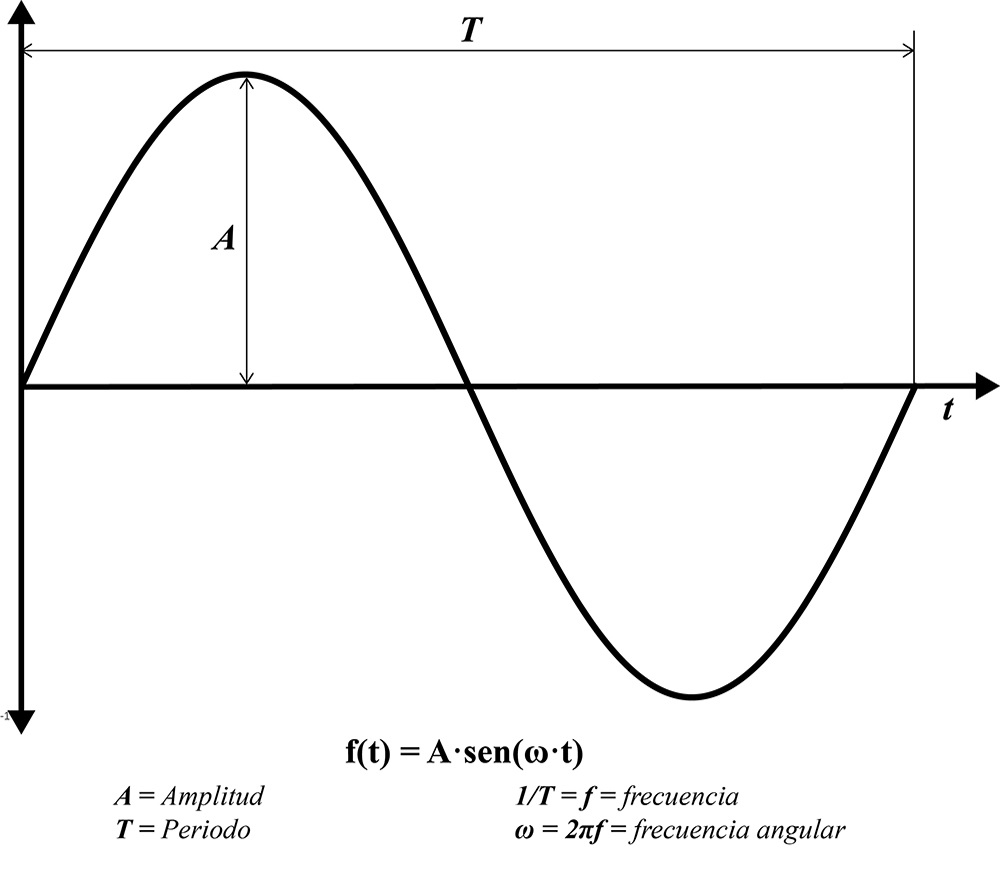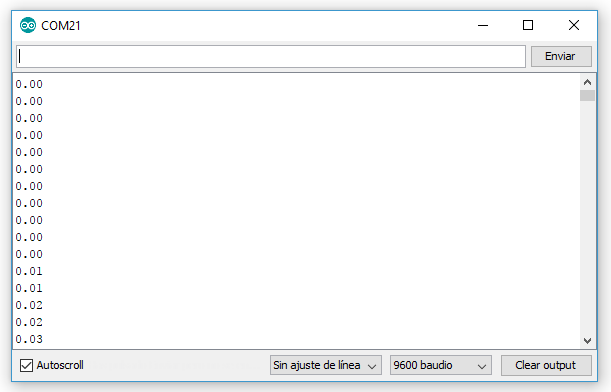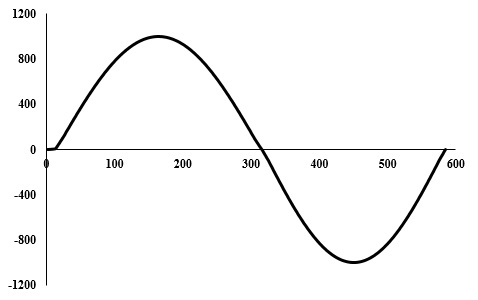# The speed of serial communication on Arduino

0
30757Serial communication based on the RS-232 protocol is the main form of communication that Arduino has to exchange information with other devices. Through the serial port is how users can send information from the computer. The Arduino IDE uses serial communication to load the code into the Arduino.

Most people who have a little experience with Arduino are able to recognize the following instruction:

This line of code tells the Arduino to initiate communication with the computer (or any device connected to the RX and TX pins) with a serial communication rate of 9600 bits per second (baud). There are other data transmission rates, such as 300, 600, 1200, 2400, 4800, 9600, 14400, 19200, 28800, 38400, 57600, or 115200. These are the default values for communication between Arduino and a computer, which can be seen in the Serial Monitor of the Arduino IDE.

##### Testing code

To carry out the tests, I will ask the Arduino to generate the values of a sine wave for me and print them to the Serial Monitor. A sine wave has certain characteristics such as amplitude, period, frequency, and angular frequency.I will ask Arduino to generate the values of a sine wave with a period of 5 seconds. Thus, the frequency will be 0.2 Hz, and the angular frequency ω = 0.2 · 2 · π. I’m going to instruct the Arduino to start generating values from the sine function from 5000 milliseconds, up to 10000 milliseconds. Thus, the result should be a single period sine wave. An amplitude of 1000 and a serial communication speed of 9600 baud will be considered.

This code generates values between 5 and 10 seconds after execution starts. The following results are seen on the serial monitor:This algorithm will generate a total of 585 values. These 585 values plotted in Excel describe a graph of a sine wave, as shown in the following image.If this algorithm is repeated several times, 585 values are always generated. Now, how about we vary the speed of the port? Running the algorithm using a 4800 baud rate yields 297 values, that is, slightly less than half of the values obtained when 9600 baud is used.

When you use 19200 baud, that is, twice the 9600, you get 1158 values. This is slightly less than double the values you get when using 9600 baud rate. The following graph shows the number of values generated as a function of serial port speeds.

A more or less linear behavior. Increasing the speed of serial communication allows you to take advantage of more work cycles of the Arduino and, in turn, generate more data. The same thing happens when data is sent to the Arduino, just as I once explained in this post.

The generated algorithm will produce a value of the sine wave with each cycle in which the Arduino repeats the execution of the instruction. This happens at a higher speed than the Arduino can print the data on the Serial Port. If you increase the speed to the maximum (115200 baud) you get the most amount of printed data generated, although in reality the Arduino is capable of producing more than 7022 data in 5 seconds.

The problem is that storing this data requires memory space, so it will not be possible to store all the data generated for later printing. Printing in “real time” is slower, but does not consume a lot of memory space. Basically the Arduino frees the memory space allocated to the calculation of the wave value in each cycle of the while structure, which keeps the SRAM memory with enough space available.

hat said, I hope the information presented is useful to you. Feel free to send me your questions or comments on this topic.